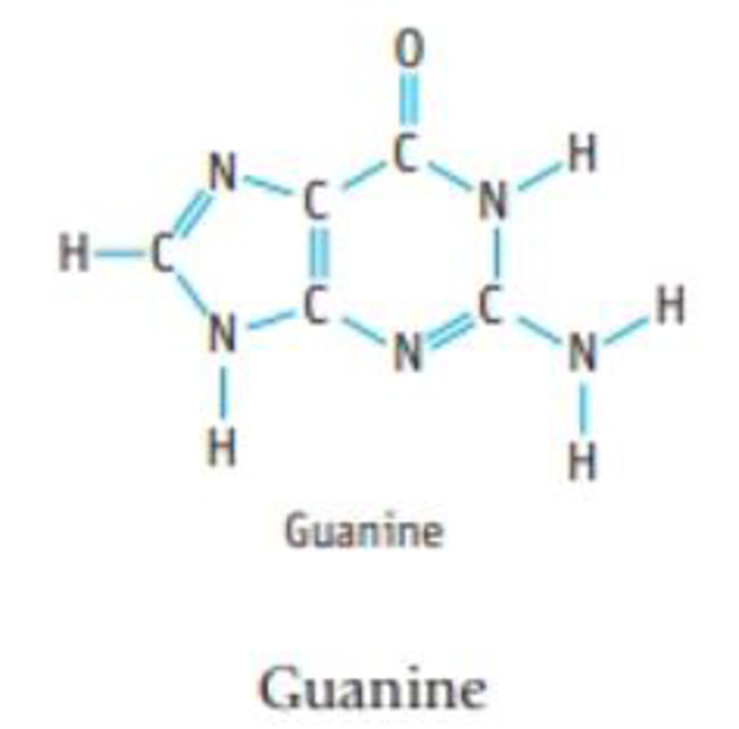Chapter 8, Problem 93IL

Chapter
Section
Textbook Problem

Guanine is present in both DNA and RNA. (a) What is the most polar bond in the molecule? (b) What is the N-C=N angle in the 6-member ring? (c) What is the N-C=N angle in the 5-member ring? (d) What is the bond angle around the N atom of the NH3 group?(a)

Interpretation Introduction

Interpretation:

The most polar bond in the molecule has to be determined.

Concept introduction:

Polar covalent bond involves an unequal sharing of electrons between two bonded atoms; it creates a partial separation of charge and a dipole moment.

Covalent bonds are polar, if there is a difference in electronegativity between the bonded atoms.

Explanation

The given compound is Guanine

The electronegativity of atoms in a bond will be the deciding factor to predict whether the bond is polar or non-polar.

Electronegativities of the atoms in Guanine are given below:

Oxygen3.5Carbon2.5Nitrogen3.0Hydrogen2.1

Electronegativity differences between bonds are given below:

C-O=3.5-2

(b)

Interpretation Introduction

Interpretation:

The NC=N angle in the six member ring has to be determined.

Concept introduction:

• In VSEPR, the geometry of the molecule is explained based on minimizing electrostatic repulsion between the molecules’ valence electrons around a central atom
• Bond angle is the angle between two bonds of a molecule and it is determined based on the electron-domain geometry.

[Bond angles: tetrahedral = 109.5o, trigonal planar = 120o, T-shape = 90o]

Electron geometry is the shape of a molecule predicted by considering both bond electron pairs and lone pair of electrons.

Molecular geometry is the shape of a molecule predicted by considering only bond pair of electrons.

(c)

Interpretation Introduction

Interpretation:

The NC=N angle in the five member ring has to be determined.

Concept introduction:

• In VSEPR, the geometry of the molecule is explained based on minimizing electrostatic repulsion between the molecules’ valence electrons around a central atom
• Bond angle is the angle between two bonds of a molecule and it is determined based on the electron-domain geometry.

[Bond angles: tetrahedral = 109.5o, trigonal planar = 120o, T-shape = 90o]

Electron geometry is the shape of a molecule predicted by considering both bond electron pairs and lone pair of electrons.

Molecular geometry is the shape of a molecule predicted by considering only bond pair of electrons.

(d)

Interpretation Introduction

Interpretation:

The bond angle around the nitrogen atom of NH2 has to be determined.

Concept introduction:

• In VSEPR, the geometry of the molecule is explained based on minimizing electrostatic repulsion between the molecules’ valence electrons around a central atom
• Bond angle is the angle between two bonds of a molecule and it is determined based on the electron-domain geometry.

[Bond angles: tetrahedral = 109.5o, trigonal planar = 120o, T-shape = 90o]

Electron geometry is the shape of a molecule predicted by considering both bond electron pairs and lone pair of electrons.

Molecular geometry is the shape of a molecule predicted by considering only bond pair of electrons.

Still sussing out bartleby?

Check out a sample textbook solution.

See a sample solution

The Solution to Your Study Problems

Bartleby provides explanations to thousands of textbook problems written by our experts, many with advanced degrees!

Get Started